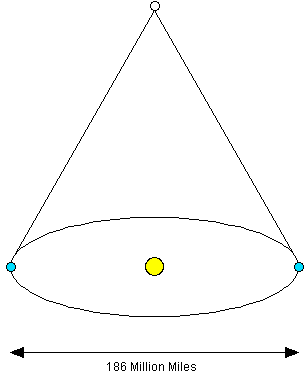# Parallax• Apparent shift of foreground objects with respect to the background due to a change in perspective
• Used to determine the distance of stars from the Earth
• Provides proof that the Earth orbits the Sun

## Formula

• $D = \frac{1}{p}$
• $D$ is distance in parsecs
• $p$ is the parallax angle in arcseconds